moveit2 The MoveIt Motion Planning Framework for ROS 2.
trajectory_processing Namespace Reference

## Classes

class  RuckigSmoothing

class  PathSegment

class  Path

class  Trajectory

class  TimeOptimalTrajectoryGeneration

class  TimeParameterization

class  LinearPathSegment

class  CircularPathSegment

## Enumerations

enum  LimitType { VELOCITY , ACCELERATION }

## Functions

MOVEIT_CLASS_FORWARD (TimeOptimalTrajectoryGeneration)

bool totgComputeTimeStamps (const size_t num_waypoints, robot_trajectory::RobotTrajectory &trajectory, const double max_velocity_scaling_factor=1.0, const double max_acceleration_scaling_factor=1.0)
Compute a trajectory with the desired number of waypoints. Resampling the trajectory doesn't change the start and goal point, and all re-sampled waypoints will be on the path of the original trajectory (within path_tolerance_). However, controller execution is separate from MoveIt and may deviate from the intended path between waypoints. path_tolerance_ is defined in configuration space, so the unit is rad for revolute joints, meters for prismatic joints. This is a free function because it needs to modify the const resample_dt_ member of TimeOptimalTrajectoryGeneration class. More...

MOVEIT_CLASS_FORWARD (TimeParameterization)
Base class for trajectory parameterization algorithms. More...

bool isTrajectoryEmpty (const moveit_msgs::msg::RobotTrajectory &trajectory)
Checks if a robot trajectory is empty. More...

std::size_t trajectoryWaypointCount (const moveit_msgs::msg::RobotTrajectory &trajectory)
Returns the number of waypoints in a robot trajectory. More...

bool applyTOTGTimeParameterization (robot_trajectory::RobotTrajectory &trajectory, double velocity_scaling_factor, double acceleration_scaling_factor, double path_tolerance=0.1, double resample_dt=0.1, double min_angle_change=0.001)
Applies time parameterization to a robot trajectory using the Time-Optimal Trajectory Generation (TOTG) algorithm. More...

bool applyRuckigSmoothing (robot_trajectory::RobotTrajectory &trajectory, double velocity_scaling_factor, double acceleration_scaling_factor, bool mitigate_overshoot=false, double overshoot_threshold=0.01)
Applies Ruckig smoothing to a robot trajectory. More...

## Variables

const std::unordered_map< LimitType, std::string > LIMIT_TYPES

## ◆ LimitType

Enumerator
VELOCITY
ACCELERATION

Definition at line 48 of file time_optimal_trajectory_generation.h.

## ◆ applyRuckigSmoothing()

 bool trajectory_processing::applyRuckigSmoothing ( robot_trajectory::RobotTrajectory & trajectory, double velocity_scaling_factor, double acceleration_scaling_factor, bool mitigate_overshoot = `false`, double overshoot_threshold = `0.01` )

Applies Ruckig smoothing to a robot trajectory.

Parameters
 [in,out] trajectory The robot trajectory to be smoothed. [in] velocity_scaling_factor The factor by which to scale the maximum velocity of the trajectory. [in] acceleration_scaling_factor The factor by which to scale the maximum acceleration of the trajectory. [in] mitigate_overshoot Whether to mitigate overshoot during smoothing (default: false). [in] overshoot_threshold The maximum allowed overshoot during smoothing (default: 0.01).
Returns
True if smoothing was successful, false otherwise.

Definition at line 58 of file trajectory_tools.cpp.

Here is the call graph for this function: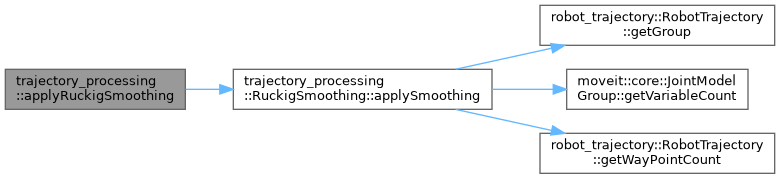Here is the caller graph for this function: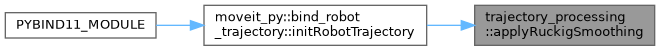## ◆ applyTOTGTimeParameterization()

 bool trajectory_processing::applyTOTGTimeParameterization ( robot_trajectory::RobotTrajectory & trajectory, double velocity_scaling_factor, double acceleration_scaling_factor, double path_tolerance = `0.1`, double resample_dt = `0.1`, double min_angle_change = `0.001` )

Applies time parameterization to a robot trajectory using the Time-Optimal Trajectory Generation (TOTG) algorithm.

Parameters
 [in,out] trajectory The robot trajectory to be time parameterized. [in] velocity_scaling_factor The factor by which to scale the maximum velocity of the trajectory. [in] acceleration_scaling_factor The factor by which to scale the maximum acceleration of the trajectory. [in] path_tolerance The path tolerance to use for time parameterization (default: 0.1). [in] resample_dt The time step to use for time parameterization (default: 0.1). [in] min_angle_change The minimum angle change to use for time parameterization (default: 0.001).
Returns
True if time parameterization was successful, false otherwise.

Definition at line 51 of file trajectory_tools.cpp.

Here is the call graph for this function: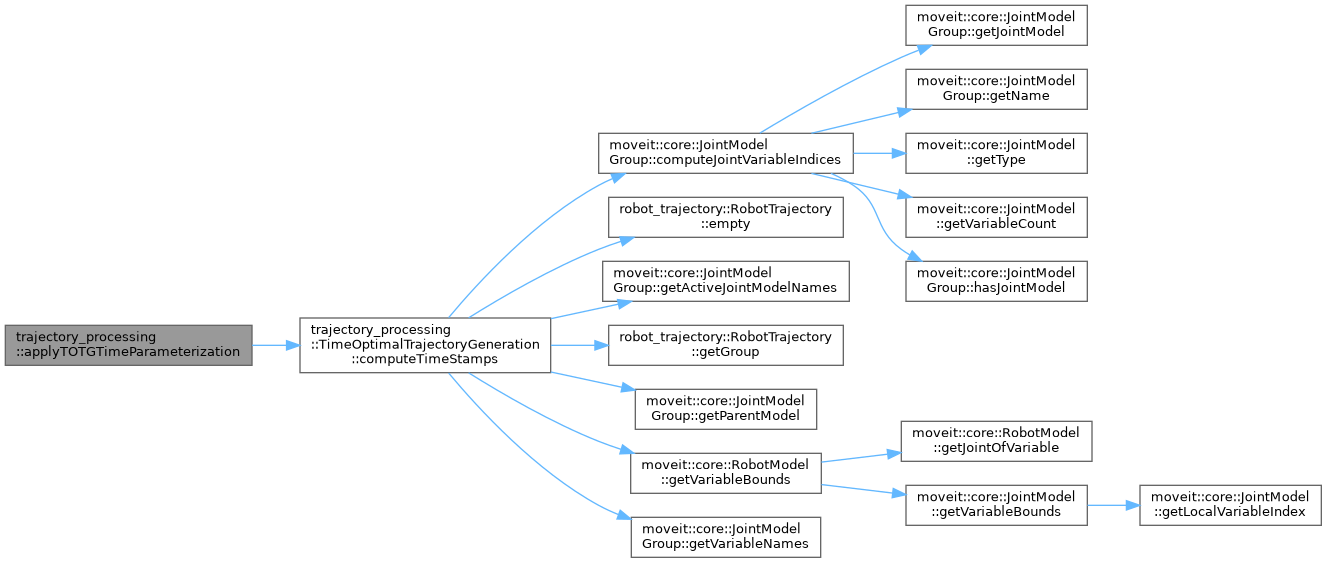Here is the caller graph for this function: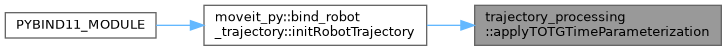## ◆ isTrajectoryEmpty()

 bool trajectory_processing::isTrajectoryEmpty ( const moveit_msgs::msg::RobotTrajectory & trajectory )

Checks if a robot trajectory is empty.

Parameters
 [in] trajectory The robot trajectory to check.
Returns
True if the trajectory is empty, false otherwise.

Definition at line 42 of file trajectory_tools.cpp.

## ◆ MOVEIT_CLASS_FORWARD() [1/2]

 trajectory_processing::MOVEIT_CLASS_FORWARD ( TimeOptimalTrajectoryGeneration )

## ◆ MOVEIT_CLASS_FORWARD() [2/2]

 trajectory_processing::MOVEIT_CLASS_FORWARD ( TimeParameterization )

Base class for trajectory parameterization algorithms.

## ◆ totgComputeTimeStamps()

 bool trajectory_processing::totgComputeTimeStamps ( const size_t num_waypoints, robot_trajectory::RobotTrajectory & trajectory, const double max_velocity_scaling_factor = `1.0`, const double max_acceleration_scaling_factor = `1.0` )

Compute a trajectory with the desired number of waypoints. Resampling the trajectory doesn't change the start and goal point, and all re-sampled waypoints will be on the path of the original trajectory (within path_tolerance_). However, controller execution is separate from MoveIt and may deviate from the intended path between waypoints. path_tolerance_ is defined in configuration space, so the unit is rad for revolute joints, meters for prismatic joints. This is a free function because it needs to modify the const resample_dt_ member of TimeOptimalTrajectoryGeneration class.

Parameters
 num_waypoints The desired number of waypoints (plus or minus one due to numerical rounding). [in,out] trajectory A path which needs time-parameterization. It's OK if this path has already been time-parameterized; this function will re-time-parameterize it. max_velocity_scaling_factor A factor in the range [0,1] which can slow down the trajectory. max_acceleration_scaling_factor A factor in the range [0,1] which can slow down the trajectory.

Definition at line 1090 of file time_optimal_trajectory_generation.cpp.

Here is the caller graph for this function: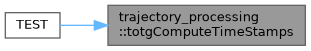## ◆ trajectoryWaypointCount()

 std::size_t trajectory_processing::trajectoryWaypointCount ( const moveit_msgs::msg::RobotTrajectory & trajectory )

Returns the number of waypoints in a robot trajectory.

Parameters
 [in] trajectory The robot trajectory to count waypoints in.
Returns
The number of waypoints in the trajectory.

Definition at line 47 of file trajectory_tools.cpp.

## ◆ LIMIT_TYPES

 const std::unordered_map trajectory_processing::LIMIT_TYPES
Initial value:

Definition at line 54 of file time_optimal_trajectory_generation.h.